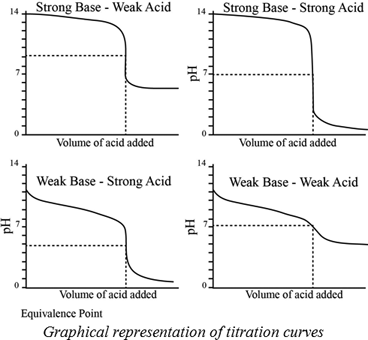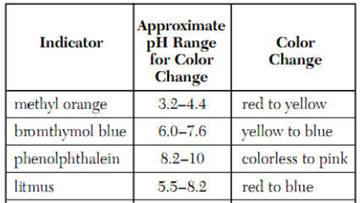# Without doing detailed calculations, sketch the curve for the titration of 50 mL of 0.050 M pyridine, C 6 H 5 N (a weak base), with 0.10 M HCl. Indicate the approximate pH at the beginning of the titration and at the equivalence point. What is the total solution volume at the equivalence point?### Chemistry & Chemical Reactivity

9th Edition
John C. Kotz + 3 others
Publisher: Cengage Learning
ISBN: 9781133949640

#### Solutions

Chapter
Section### Chemistry & Chemical Reactivity

9th Edition
John C. Kotz + 3 others
Publisher: Cengage Learning
ISBN: 9781133949640
Chapter 17, Problem 32PS
Textbook Problem
87 views

## Without doing detailed calculations, sketch the curve for the titration of 50 mL of 0.050 M pyridine, C6H5N (a weak base), with 0.10 M HCl. Indicate the approximate pH at the beginning of the titration and at the equivalence point. What is the total solution volume at the equivalence point?

Interpretation Introduction

Interpretation:

The curve for titration of 50 mL of 0.050 M  pyridine, C5H5N with 0.10 M HCl has to be sketched. The approximate pH at the beginning of the titration and at a equivalence point has to be indicated. And the total solution volume at the equivalence point has to be determined.

Concept introduction:

A titration is one of the most useful ways of determining accurately the quantity of an acid , a base or some other substances in a mixture.

The pH at the equivalence point of a strong acid –strong base titration is 7.

A weak acid titrated with a strong base leads to a pH > 7 at the equivalence point.

A weak base titrated with a strong acid leads to pH < 7 at the equivalence point.Indicators:

A chemical substances which gives a visible change  in the titration.

Some important indicators are as follow.### Explanation of Solution

Pyridine is a weak base , the estimated pH value of the solution is about pH = 9 from the exponent of its Kb value (Kb=1.4×109).

Here, we are using for titration basic solution versus acidic solution. Therefore, acidic solution will be acidic at high concentrations.

The pyridine reacts with hydrogen chloride to form pyridinium chloride. It is a weak acid. Then the solution will be acidic. So the pH can be estimated as pH = 5

### Still sussing out bartleby?

Check out a sample textbook solution.

See a sample solution

#### The Solution to Your Study Problems

Bartleby provides explanations to thousands of textbook problems written by our experts, many with advanced degrees!

Get Started

Find more solutions based on key concepts
Why should units be carried along with numbers in a calculation?

General Chemistry - Standalone book (MindTap Course List)

Water intoxication cannot occur because water is so easily excreted by the body. T F

Nutrition: Concepts and Controversies - Standalone book (MindTap Course List)

Explain the acronyms mks, SI, and cgs.

An Introduction to Physical Science

How does the CNO cycle differ from the proton-proton chain? How is it similar?

Horizons: Exploring the Universe (MindTap Course List)

(a) What is the angular speed of the second hand of an analog clock? (b) What is the direction of as you view ...

Physics for Scientists and Engineers, Technology Update (No access codes included)

What effects might be caused by global warming?

Oceanography: An Invitation To Marine Science, Loose-leaf Versin